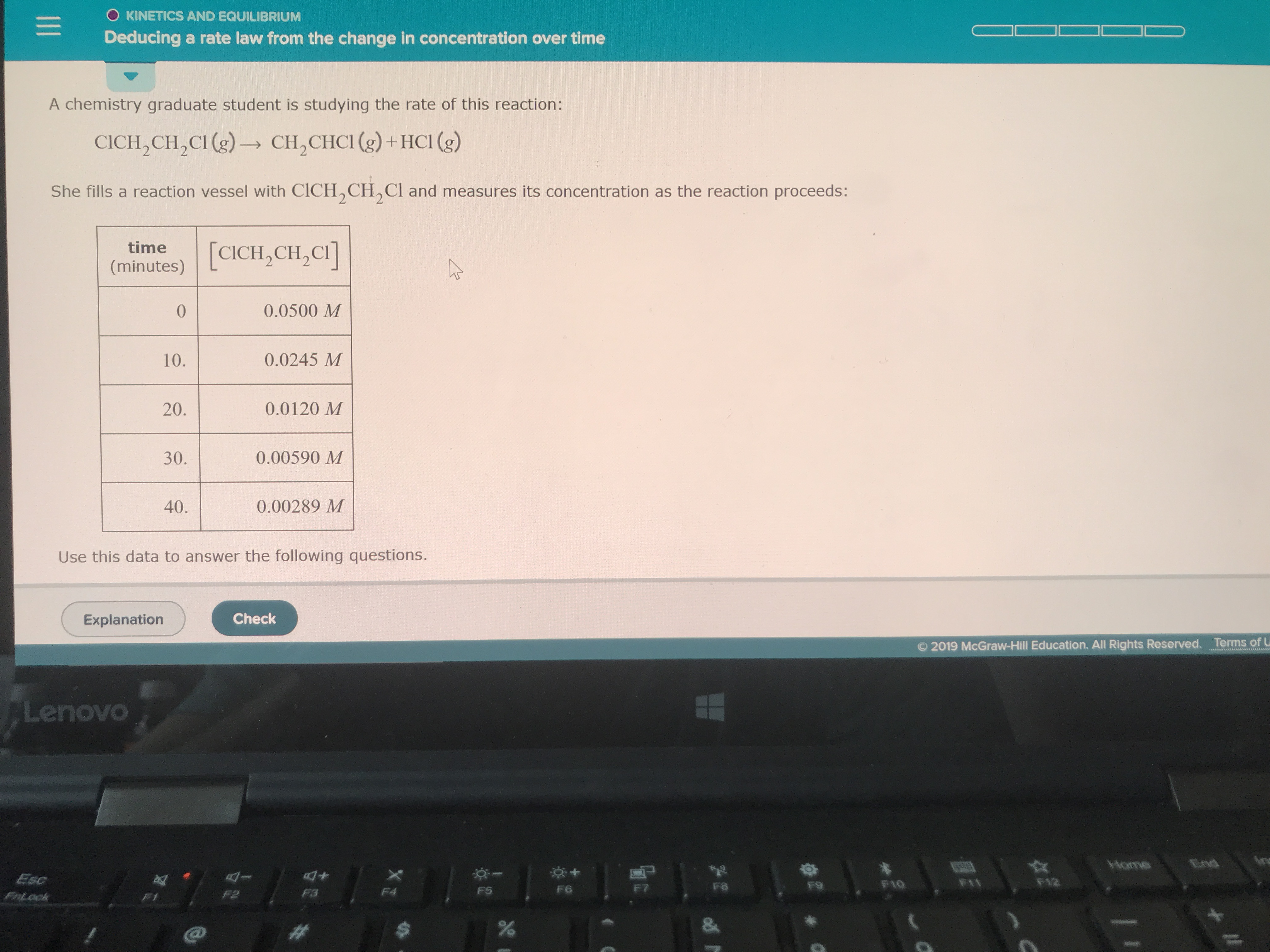# A chemistry graduate student is studying the rate of this reaction: ClCH2CH2Cl(g) → CH2CHCl(g) + HCl(g) She fills reaction vessel with ClCH2CH2Cl and measures its concentration as the reaction proceeds: Use this data to answer the following questions. Write the rate law for this reaction Rate=k [?]. Then calculate the value of the rate constant k. Round your answer to 2 significant digits.